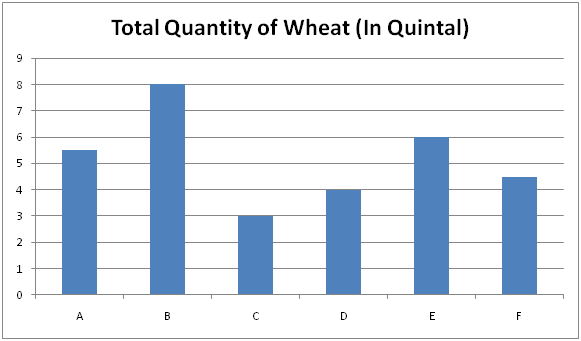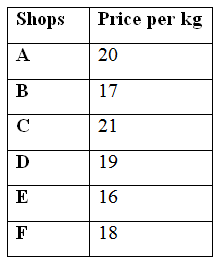# SBI PO Prelims Quantitative Aptitude Questions 2019 – Day 16

SBI PO 2019 Notification will be expected soon. It is one of the most expected recruitment among the banking aspirants. Every year the exam pattern for SBI PO has been changing. Depends upon the changing of exam pattern the questions are quite harder compare to the previous year. So the questions are in high level than the candidate’s assumption.

As per the latest trend, our IBPS Guide is providing the updated New Exam Pattern Quantitative Aptitude questions for SBI PO 2019 Day 16. Our Skilled experts were mounting the questions based on the aspirant’s needs. So candidates shall start your preparation and practice on daily basis with our SBI PO pattern quantitative aptitude questions 2019 day 16. Start your effective preparation from the right beginning to get success in upcoming SBI PO 2019.

[WpProQuiz 5279]

### Click Here for SBI PO Pre 2019 High-Quality Mocks Exactly on SBI Standard

Directions (1 – 5): What approximate value should come in the place of question mark (?) in the following questions?

1) [(24.98 × 33) ÷ 10.82 – 2398 ÷ 119.65] = 5 × ?

a) 18

b) 25

c) 11

d) 6

e) 33

2) (14.95)2 ÷ 3373 + (44.92)2 ÷ 15.13 = ? – 38416

a) 164

b) 132

c) 115

d) 88

e) 193

3) 12 5/6 % of 8504 + 18 2/3 % of 2796 + 8 1/4 % of 9697 = ?

a) 1167

b) 2785

c) 3352

d) 3616

e) 2413

4) 3 4/5 + 7 8/9 – 5 1/6 – 3 1/3 = ? – 6 3/4

a) 23

b) 5

c) 34

d) 11

e) 42

5) (80.78)2 × (6561)1/4 × (36.11)2 ÷ (38 × (12.19)2) = 3?

a) 5

b) 4

c) 7

d) 8

e) 6

Directions (6 – 10): Study the following graph carefully and answer the given question.

The following bar graph shows the total quantity of sale of wheat (In Quintal) of 6 different shops and the table shows the price per kg of wheat.Note: 1 Quintal = 100 kg

Total amount earned = Total Quantity of Wheat sold * Price per kg

6) Total Quantity of Wheat sold by the shop A and C together is approximately what percentage of total quantity of Wheat sold by the shop B and D together?

a) 50 %

b) 85 %

c) 115 %

d) 70 %

e) 100 %

7) Find the ratio between the total amount earned by shop B to that of shop E?

a) 17 : 12

b) 23 : 28

c) 11 : 15

d) 8 : 3

e) None of these

8) Find the total amount earned by shop D, E and F together?

a) 20800

b) 22600

c) 25300

d) 27500

e) None of these

9) The total amount earned by shop B is approximately what percentage more than the total amount earned by shop C?

a) 95 %

b) 115 %

c) 80 %

d) 140 %

e) 60 %

10) Find the difference between the total quantity of wheat sold by the shop A, B and E together to that of the shop C, D and F together?

a) 10 quintal

b) 15 quintal

c) 17 quintal

d) 8 quintal

e) None of these

Direction (1-5) :

[(25 × 33) ÷ 11 – 2400 ÷ 120] = 5x

(25*33)/11 – (2400/120) = 5x

75 – 20 = 5x

5x = 55

X = 11

(15)2 ÷ ∛3375 + (45)2 ÷ 15 = x – ∜38416

(15*15)/15 + (45*45)/15 = x – 14

X = 15 + 135 + 14 = 164

13 % of 8500 + 19 % of 2800 + 8 % of 9700 = x

X = (13/100)*8500 + (19/100)*2800 + (8/100)*9700

X = 1105 + 532 + 776 = 2413

3 4/5 + 7 8/9 – 5 1/6 – 3 1/3 + 6 ¾ = x

X = 4 + 8 – 5 – 3 + 7 = 11

(81)2 × (6561)1/4 × (36)2 ÷ (38 × (12)2) = 3x

(81*81*9*36*36)/(38*12*12) = 3x

(9*3*3) = 3x

3x = 34 (Base value equal. So, Power value also equal)

X = 4

Direction (6-10) :

Total Quantity of Wheat sold by the shop A and C together

= > 5.5 + 3 = 8.5 quintal

Total quantity of Wheat sold by the shop B and D together

= > 8 + 4 = 12 quintal

Required % = (8.5/12)*100 = 70 %

The total amount earned by shop B

= > (800*17) = 13600

The total amount earned by shop E

= > (600*16) = 9600

Required ratio = 13600 : 9600 = 17 : 12

The total amount earned by shop D, E and F together

= > [(400*19) + (600*16) + (450*18)]

= > [7600 + 9600 + 8100]

= > 25300

The total amount earned by shop B

= > 800*17 = 13600

The total amount earned by shop C

= > 300*21 = 6300

Required % = [(13600 – 6300)/6300]*100 = 115 %

The total quantity of wheat sold by the shop A, B and E together

= > 5.5 + 8 + 6 = 19.5 quintal

The total quantity of wheat sold by the shop C, D and F together

= > 3 + 4 + 4.5 = 11.5 quintal

Required difference = 19.5 – 11.5 = 8 quintal